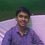# Help me!

Let $P(x) = (x-1)(x-2)(x-3)$.

Find for how many polynomials $Q(x)$ does there exist a polynomial $R(x)$ of degree 3 such that $P(Q(x)) = P(x) \cdot R(x)$.Note by Vedant Sharda
5 years, 4 months ago

This discussion board is a place to discuss our Daily Challenges and the math and science related to those challenges. Explanations are more than just a solution — they should explain the steps and thinking strategies that you used to obtain the solution. Comments should further the discussion of math and science.

When posting on Brilliant:

• Use the emojis to react to an explanation, whether you're congratulating a job well done , or just really confused .
• Ask specific questions about the challenge or the steps in somebody's explanation. Well-posed questions can add a lot to the discussion, but posting "I don't understand!" doesn't help anyone.
• Try to contribute something new to the discussion, whether it is an extension, generalization or other idea related to the challenge.

MarkdownAppears as
*italics* or _italics_ italics
**bold** or __bold__ bold
- bulleted- list
• bulleted
• list
1. numbered2. list
1. numbered
2. list
Note: you must add a full line of space before and after lists for them to show up correctly
paragraph 1paragraph 2

paragraph 1

paragraph 2

[example link](https://brilliant.org)example link
> This is a quote
This is a quote
    # I indented these lines
# 4 spaces, and now they show
# up as a code block.

print "hello world"
# I indented these lines
# 4 spaces, and now they show
# up as a code block.

print "hello world"
MathAppears as
Remember to wrap math in $$ ... $$ or $ ... $ to ensure proper formatting.
2 \times 3 $2 \times 3$
2^{34} $2^{34}$
a_{i-1} $a_{i-1}$
\frac{2}{3} $\frac{2}{3}$
\sqrt{2} $\sqrt{2}$
\sum_{i=1}^3 $\sum_{i=1}^3$
\sin \theta $\sin \theta$
\boxed{123} $\boxed{123}$

Sort by:

What are your thoughts? What have you tried?

Must the polynomial have complex, real or integer coefficients?

Staff - 5 years, 4 months ago

What I have tried is :

Degree of P(x) is 3 and R(x) is also 3

So degree of P(x).R(x) is 6.

Degree of Q(x) must be 2 so that degree of P(Q(x)) is 6.

P(Q(x)) = P(x).R(x)

(Q(x)-1)(Q(x)-2)(Q(x)-3) = (x-1)(x-2)(x-3).R(x)

Now what I have to do ?

- 5 years, 3 months ago

Great job. A key piece of information is that the degree of Q(x) is 6.

What can we say about the value of $Q(1)$? Q(2)? Q(3)?

Staff - 5 years, 3 months ago

To find Q(1) , put x=1 in

(Q(x)-1)(Q(x)-2)(Q(x)-3) = (x-1)(x-2)(x-3).R(x)

[Q(1)-1][Q(1)-2][Q(1)-3] =0

Q(1)-1 = 0. So Q(1) =1

Q(1)-2 = 0. So Q(1) = 2

Q(1)-3 = 0. So Q(1) = 3

Hence Q(1) = 1or 2or 3

Similarly Q(2) = 1or 2or 3

And Q(3) = 1or 2or 3

- 5 years, 3 months ago

Great, so we now know the possible values of $Q(1), Q(2), Q(3)$.

Lets say that $Q(1) = 1, Q(2) = 2, Q(3) = 2$. How many polynomials $Q(x)$ of degree 2 satisfy that condition?

Staff - 5 years, 3 months ago

I can't get it

- 5 years, 3 months ago

Check out lagrange interpolation

Hint: If we have $n$ equations of the form $f(x_i) = y _ i$, then there is a unique polynomial of degree at most $n-1$ which satisfies the conditions.

Staff - 5 years, 3 months ago

I think the answer is 2

- 5 years, 4 months ago

- 5 years, 3 months ago

Yup, that's the numerical answer. The more interesting part is the actual solution.

Staff - 5 years, 3 months ago

Let $Q(x) = ax^2+bx + c$ and put that into P. Plug in x =1,2,3 and now you have a system of three linear equations with three possibilities each. Now it's just combinatorics! Calculate the inverse matrix and it is easy to see that out of the 27, 5 do not work. This the answer is 22.

- 5 years, 3 months ago